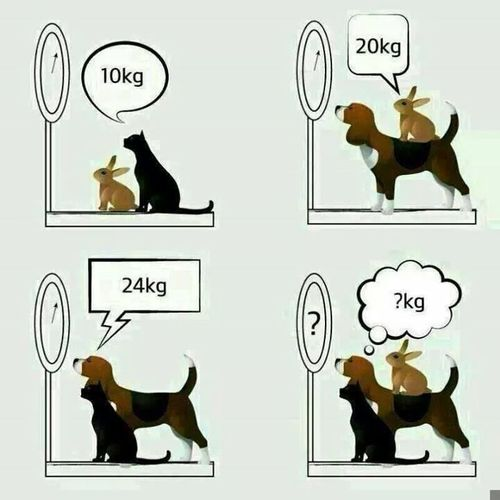The cat and the rabbit weigh 10kg. The dog and the rabbit weigh 20kg. The dog and the cat weigh 24kg. What is the total weight of the dog, cat and rabbit?The total weight of the dog, cat and rabbit is 27kg.

The dog, cat and rabbit weigh 17kg, 7kg and 3kg respectively.

Let D=Dog, C=Cat, R=Rabbit. We can come with the following equations:
C + R = 10
D + R = 20
D + C = 24

From 1st equation, we get: R = 10 -C

Substitute that into 2nd equation and we get: D + (10 – C) = 20

Solving for D gives: D = C + 10

Substitute that into 3rd equation and we get: (C + 10) + C = 24

Solving for C gives 7. We can then substitute C=7 in the other equations to get the weight of D and R.

Next Riddle »